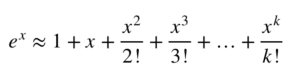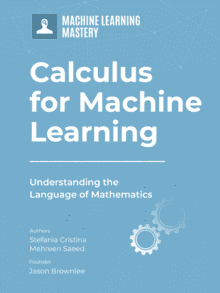# A Gentle Introduction to Taylor Series

Last Updated on March 16, 2022

A Gentle Introduction to Taylor Series

Taylor series expansion is an awesome concept, not only the world of mathematics, but also in optimization theory, function approximation and machine learning. It is widely applied in numerical computations when estimates of a function’s values at different points are required.

In this tutorial, you will discover Taylor series and how to approximate the values of a function around different points using its Taylor series expansion.

After completing this tutorial, you will know:

• Taylor series expansion of a function
• How to approximate functions using Taylor series expansion

Let’s get started.A Gentle Introduction To Taylor Series. Photo by Muhammad Khubaib Sarfraz, some rights reserved.

## Tutorial Overview

This tutorial is divided into 3 parts; they are:

1. Power series and Taylor series
2. Taylor polynomials
3. Function approximation using Taylor polynomials

## What Is A Power Series?

The following is a power series about the center x=a and constant coefficients c_0, c_1, etc.## What Is A Taylor Series?

It is an amazing fact that functions which are infinitely differentiable can generate a power series called the Taylor series. Suppose we have a function f(x) and f(x) has derivatives of all orders on a given interval, then the Taylor series generated by f(x) at x=a is given by:The second line of the above expression gives the value of the kth coefficient.

If we set a=0, then we have an expansion called the Maclaurin series expansion of f(x).

### Want to Get Started With Calculus for Machine Learning?

Take my free 7-day email crash course now (with sample code).

Click to sign-up and also get a free PDF Ebook version of the course.

## Examples Of Taylor Series Expansion

Taylor series generated by f(x) = 1/x can be found by first differentiating the function and finding a general expression for the kth derivative.The Taylor series about various points can now be found. For example:## Taylor Polynomial

A Taylor polynomial of order k, generated by f(x) at x=a is given by:For the example of f(x)=1/x, the Taylor polynomial of order 2 is given by:## Approximation via Taylor Polynomials

We can approximate the value of a function at a point x=a using Taylor polynomials. The higher the order of the polynomial, the more the terms in the polynomial and the closer the approximation is to the actual value of the function at that point.

In the graph below, the function 1/x is plotted around the point x=1 (left) and x=3 (right). The line in green is the actual function f(x)= 1/x. The pink line represents the approximation via an order 2 polynomial.

## More Examples of Taylor Series

Let’s look at the function g(x) = e^x.  Noting the fact that the kth order derivative of g(x) is also g(x), the expansion of g(x) about x=a, is given by:Hence, around x=0, the series expansion of g(x) is given by (obtained by setting a=0):The polynomial of order k generated for the function e^x around the point x=0 is given by:The plots below show polynomials of different orders that estimate the value of e^x around x=0. We can see that as we move away from zero, we need more terms to approximate e^x more accurately. The green line representing the actual function is hiding behind the blue line of the approximating polynomial of order 7.

## Taylor Series In Machine Learning

A popular method in machine learning for finding the optimal points of a function is the Newton’s method. Newton’s method uses the second order polynomials to approximate a function’s value at a point. Such methods that use second order derivatives are called second order optimization algorithms.

## Extensions

This section lists some ideas for extending the tutorial that you may wish to explore.

• Newton’s method
• Second order optimization algorithms

If you explore any of these extensions, I’d love to know. Post your findings in the comments below.

This section provides more resources on the topic if you are looking to go deeper.

## Summary

In this tutorial, you discovered what is Taylor series expansion of a function about a point. Specifically, you learned:

• Power series and Taylor series
• Taylor polynomials
• How to approximate functions around a value using Taylor polynomials

## Get a Handle on Calculus for Machine Learning!#### Feel Smarter with Calculus Concepts

...by getting a better sense on the calculus symbols and terms

Discover how in my new Ebook:
Calculus for Machine Learning

It provides self-study tutorials with full working code on:
differntiation, gradient, Lagrangian mutiplier approach, Jacobian matrix, and much more...

### 4 Responses to A Gentle Introduction to Taylor Series

1.Satybir Singh Satybir Singh Teotia August 21, 2021 at 12:42 pm #

Wonderful explanation. Only now I could understand the concept of Taylor series.

•Adrian Tam August 23, 2021 at 4:42 am #

Thank you. Glad you like it.

2.sarva ramana May 18, 2022 at 3:27 pm #

explanation is in a way which is easy to understand for a beginner. Thanks.
Also, let us know about any of your posts/tutorials on approximating functions around certain values, with applications in Machine learning. ( with lot of examples )

•James Carmichael May 19, 2022 at 6:30 am #

Thank you for the great feedback Sarva!